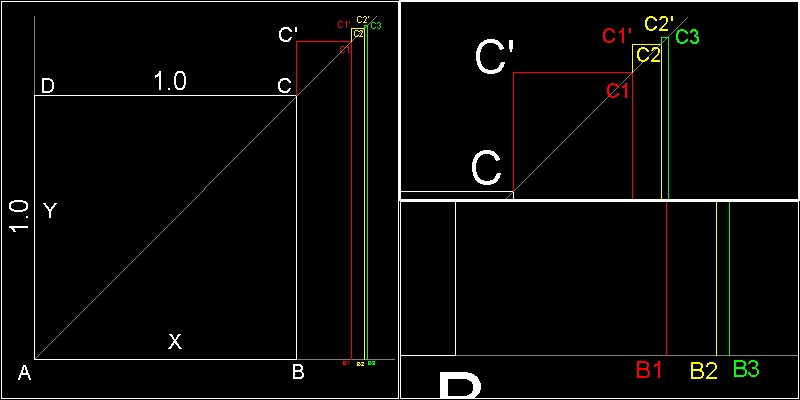Squaring the Circle
Go to   Fun_Math Content Table   Three Famous Problems   Greek Circle Squarer   Later Squarer

### Later Circle Squarers

#### René Descartes

René Descartes (1596 - 1650) ,the famous founder of analytical geometry, also used polygon approach to determine the value of p. But instead of computing the perimeters of polygons inscribing (and/or) circumscribing a given circle, he kept the perimeter length constant, and doubled its sides until the polygon approaches the circle.``` From the figure, the perimeter of the n-sided polygon (L) is L = n.AB = 2 n r tan(q/2) (1) Then after doubling the sides k times ( 2k) L = 2 n 2k r tan((q/2)/2k) = n r 2k+1 tan(q/2k+1) (2) Descartes started from n = 4, and q= p/2 So L = 4 r 2k+1 tan((p/2)/2k+1) = (2r) 2k+2 tan(p/2k+2) Dividing both sides by 2r(Diameter), we get L/(2r) = 2k+2 tan(p/2k+2) (3) The left hand side is the ratio of perimeter to diameter. Therefore as k becomes large, this will approach the value of p. Or p is a limit value of 2k+2 tan(p/2k+2) (4) ```

******** Descartes_pi_model_base.dwg ********

#### Graphical solution of René Descartes******** Descartes_circle_square_model.dwg ********

 ``` From (3) xk = 2r = (L/2k+2)cot(p/2k+2) (5) Descartes noticed that (5) is the positive root of the following recursive equation. xk(xk - xk-1) = x02/4k (6) where L = 4x0 When xk-1 is given, this is a quadratic equation with respect to xk. This can be verified by substituting Xk, and xk-1 expressed in terms of cot(q) into (6) and using the double angle formula for cotangent,i.e. cot(2q) = (cot2q - 1)/(2 cotq) The graphical interpretation of (6) is as follows: Start with a square ABCD with a unit side length. Find points B1,C1 such that area B B1 C1 C' is 1/4 of the area of the square. Next find points B2, C2 which makes the area B1 B2 C2 C1' equal to 1/4 of rectangle B B1 C1 C'. And so on ... During the process, length ABi, where i = 1,2,3,...., is the diameter of the circumscribing polygon which makes the perimeter value the constant (4 x0). Therefore the estimated value of p at that level of side doubling is obtained by dividing 4 by this diameter value. ```

#### Result of René Descartes***************** Descartes_circle_square_desc.dwg *****************

To create this drawing :
Then from command line, type Descartes_pi

 ``` The result of this program is shown below: 15-th decimal place is correct after 25-th step. step Approximate value 1 3.31370 84989 84761 2 3.18259 78780 74528 3 3.15172 49074 29256 4 3.14411 83852 45905 5 3.14222 36299 42458 6 3.14175 03691 68967 7 3.14163 20807 03182 8 3.14160 25102 56809 9 3.14159 51177 49589 10 3.14159 32696 29307 11 3.14159 28075 99644 12 3.14159 26920 92255 13 3.14159 26632 15408 14 3.14159 26559 96197 15 3.14159 26541 91394 16 3.14159 26537 40194 17 3.14159 26536 27393 18 3.14159 26535 99193 19 3.14159 26535 92143 20 3.14159 26535 90380 21 3.14159 26535 89939 22 3.14159 26535 89829 23 3.14159 26535 89801 24 3.14159 26535 89795 25 3.14159 26535 89793 --> Correct to 15-th decimal place ```

#### References

Go to   Fun_Math Content Table   Three Famous Problems   Greek Circle Squarer   Later Squarer

All questions/suggestions should be sent to Takaya Iwamoto

Last Updated Feb 17,2007